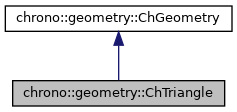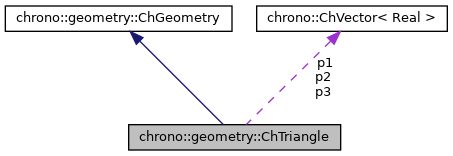chrono::geometry::ChTriangle Class Reference

## Description

A triangle geometric shape for collisions and visualization.

#include <ChTriangle.h>

Inheritance diagram for chrono::geometry::ChTriangle:[legend]
Collaboration diagram for chrono::geometry::ChTriangle:[legend]

## Public Member Functions

ChTriangle (const ChVector<> &mp1, const ChVector<> &mp2, const ChVector<> &mp3)

ChTriangle (const ChTriangle &source)

virtual ChTriangleClone () const override
"Virtual" copy constructor (covariant return type).

ChTriangleoperator= (const ChTriangle &source)
Assignment operator: copy from another triangle.

virtual GeometryType GetClassType () const override
Get the class type as unique numerical ID (faster than using ChronoRTTI mechanism). More...

virtual void GetBoundingBox (double &xmin, double &xmax, double &ymin, double &ymax, double &zmin, double &zmax, ChMatrix33<> *Rot=NULL) const override
Compute bounding box. More...

virtual ChVector Baricenter () const override
Compute center of mass It should be overridden by inherited classes.

virtual void CovarianceMatrix (ChMatrix33<> &C) const override
Compute the 3x3 covariance matrix (only the diagonal and upper part) It should be overridden by inherited classes.

virtual int GetManifoldDimension () const override
This is a surface.

bool IsDegenerated () const

bool Normal (ChVector<> &N) const

ChVector GetNormal () const

double PointTriangleDistance (ChVector<> B, double &mu, double &mv, bool &is_into, ChVector<> &Bprojected)
Given point B, computes the distance from this triangle plane, returning also the projection of point on the plane and other infos. More...

virtual void ArchiveOUT (ChArchiveOut &marchive) override
Method to allow serialization of transient data to archives.

virtual void ArchiveIN (ChArchiveIn &marchive) override
Method to allow de-serialization of transient data from archives.Public Member Functions inherited from chrono::geometry::ChGeometry
ChGeometry (const ChGeometry &source)

virtual void InflateBoundingBox (double &xmin, double &xmax, double &ymin, double &ymax, double &zmin, double &zmax, ChMatrix33<> *Rot=NULL) const
Enlarge a previous existing bounding box. More...

virtual double Size () const
Returns the radius of the sphere which can enclose the geometry.

virtual void Update ()
Generic update of internal data. More...

## Static Public Member Functions

static double PointTriangleDistance (ChVector<> B, ChVector<> &A1, ChVector<> &A2, ChVector<> &A3, double &mu, double &mv, bool &is_into, ChVector<> &Bprojected)
Given point B and a generic triangle, computes the distance from the triangle plane, returning also the projection of point on the plane and other infos. More...

static double PointLineDistance (ChVector<> &p, ChVector<> &dA, ChVector<> &dB, double &mu, bool &is_insegment)
Calculate distance between a point p and a line identified with segment dA,dB. More...

## Public Attributes

ChVector p1
first triangle vertex

ChVector p2
second triangle vertex

ChVector p3
third triangle vertexPublic Types inherited from chrono::geometry::ChGeometry
enum  GeometryType {
NONE, SPHERE, BOX, CYLINDER,
TRIANGLE, CAPSULE, CONE, LINE,
LINE_ARC, LINE_BEZIER, LINE_CAM, LINE_PATH,
LINE_POLY, LINE_SEGMENT, ROUNDED_BOX, ROUNDED_CYLINDER,
ROUNDED_CONE, TRIANGLEMESH, TRIANGLEMESH_CONNECTED, TRIANGLEMESH_SOUP
}
Enumeration of geometric objects.

## ◆ GetBoundingBox()

 void chrono::geometry::ChTriangle::GetBoundingBox ( double & xmin, double & xmax, double & ymin, double & ymax, double & zmin, double & zmax, ChMatrix33<> * Rot = NULL ) const
overridevirtual

Compute bounding box.

If a matrix Rot is not null, it should compute bounding box along the rotated directions represented by that transformation matrix Rot. It must be overridden by inherited classes.

Reimplemented from chrono::geometry::ChGeometry.

## ◆ GetClassType()

 virtual GeometryType chrono::geometry::ChTriangle::GetClassType ( ) const
inlineoverridevirtual

Get the class type as unique numerical ID (faster than using ChronoRTTI mechanism).

Each inherited class must return an unique ID.

Reimplemented from chrono::geometry::ChGeometry.

## ◆ PointLineDistance()

 double chrono::geometry::ChTriangle::PointLineDistance ( ChVector<> & p, ChVector<> & dA, ChVector<> & dB, double & mu, bool & is_insegment )
static

Calculate distance between a point p and a line identified with segment dA,dB.

Returns distance. Also, the mu value reference tells if the nearest projection of point on line falls into segment (for mu 0...1)

Returns
the distance
Parameters
 p point to be measured dA a point on the line dB another point on the line mu parametric coord: if in 0..1 interval, projection is between dA and dB is_insegment returns true if projected point is between dA and dB

## ◆ PointTriangleDistance() [1/2]

 double chrono::geometry::ChTriangle::PointTriangleDistance ( ChVector<> B, ChVector<> & A1, ChVector<> & A2, ChVector<> & A3, double & mu, double & mv, bool & is_into, ChVector<> & Bprojected )
static

Given point B and a generic triangle, computes the distance from the triangle plane, returning also the projection of point on the plane and other infos.

Returns
the signed distance
Parameters
 B point to be measured A1 point of triangle A2 point of triangle A3 point of triangle mu returns U parametric coord of projection mv returns V parametric coord of projection is_into returns true if projection falls on the triangle Bprojected returns the position of the projected point

## ◆ PointTriangleDistance() [2/2]

 double chrono::geometry::ChTriangle::PointTriangleDistance ( ChVector<> B, double & mu, double & mv, bool & is_into, ChVector<> & Bprojected )
inline

Given point B, computes the distance from this triangle plane, returning also the projection of point on the plane and other infos.

Returns
the signed distance
Parameters
 B point to be measured mu returns U parametric coord of projection mv returns V parametric coord of projection is_into returns true if projection falls on the triangle Bprojected returns the position of the projected point

The documentation for this class was generated from the following files:
• /builds/uwsbel/chrono/src/chrono/geometry/ChTriangle.h
• /builds/uwsbel/chrono/src/chrono/geometry/ChTriangle.cpp